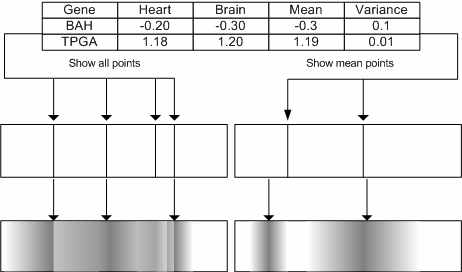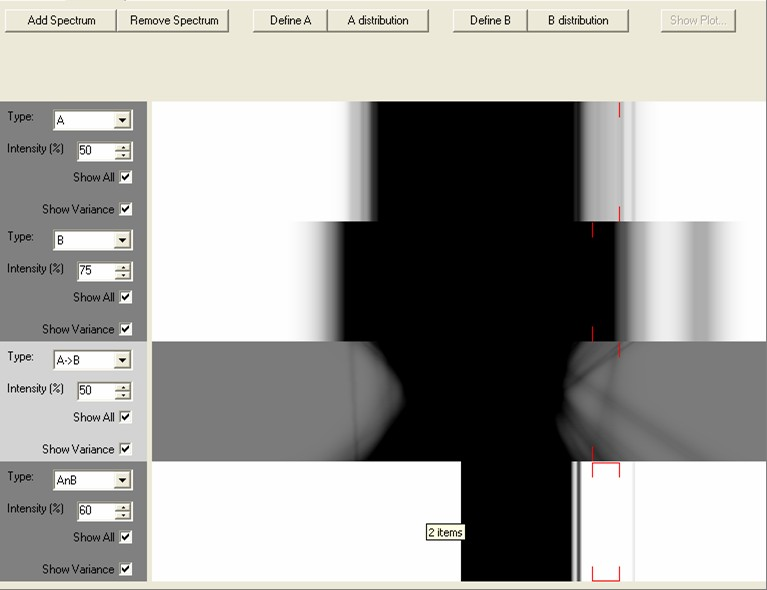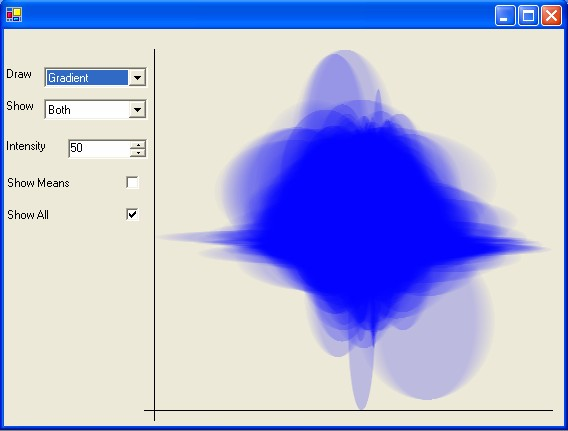# Variance Views

Two visualisations are provided to show the variance within clusters of genes, the variance can either be the standard deviation with in a cluster or can be based on a model. The two visualisations provided are:

Gene Spectrums which show the variance that occurs in a single expression experiment

Gene Clouds which can be used to comapre the variance that occurs within two experiments

## Gene Spectrums

To be able to visually compare and understand more about the fluctuations in gene expression levels the Gene Spectrum was designed and implemented. The visualisation was designed to show the values and variance for different genes within a set of experiments (see diagram below).Diagram showing how the spectrum of gene profiles can be generated based on either the mean values for a gene across all the experiments, or how they can show all the experiment gene expression values. To give an indication as to the variance that each gene shows across the experiments the values can then be blurred, using a density function. On the right hand side the resulting spectrum shows all the values, on the left-hand side only the mean values are plotted. It is also possible to plot the values from a single experiment whilst using the variance from a group of experiments.

The Gene Spectrum visualisation allows for a more uniform and compact representation of the data, providing an uncluttered display which lends itself easily to comparison and interaction tasks. To enable the spectrums to be used to either display the whole genome or a selected group of genes the intensity levels can be changed dynamically to enable examination of different parts of the spectrum. It is also possible to display results from a single gene-expression experiments, but to use a shading model (variance) from a large result set.

Two sets of genes (and associated variances/distributions) can be defined. This is done by using the associated Define A and Define B buttons to define the genes that are to be displayed, and the A distribution/B distribution buttons to define the set of experiments where the 'normal' expression values (the normal variance) are defined. Spectrums can be added or removed by selecting the Add Spectrum/Remove Spectrum buttons.

Once a spectrum has been added the Intensity can be changed to show or hide details, and the variance 'blurring' can be toggled on or off. If the Show All button is toggled off then only those genes that have been selected in another visualisation are shown (selections are shown with red lines at the top and the bottom of each spectrum). The Type of spectrum controls what each spectrum displays, these are six types of spectrums available:

• A: Displays the values for the genes defined in the A set. The variance values (if displayed) are calculated using the experiments in the A distribution set.
• B:Displays the values for the genes defined in the B set. The variance values (if displayed) are calculated using the experiments in the B distribution set.
• A->B: Shows changes between the A and B expression profiles by changing the spectrum to plot two sets of gene expression experiment. The expression values and variances for the first set of genes (A) are plotted on the top and these are combined with the second set of variances and values (B) on the bottom. If multiple expression values are being plotted for one gene then matching is done by matching the genes by using the minimum distance.
• B->A: This displays the values for the B set on the top, and the A set on the bottom.
• AnB: This subtracts the A spectrum from the B spectrum. This is useful for comparing two sets of experiments and finding areas (possible) experimental errors. The dark areas are those where there is a substantially high proportion of expression B genes than A.
• AuB: This combines the A and B spectrums into one display.When changing the experiments in the A and B sets and generated spectrums will not be updated (so it is possible to generate any number of spectrums for different sets of experiments). Any selections in a spectrum are echoed in all other spectrums and any open visualisations.

## Gene Clouds

To provide a macro-view of the variance between two sets of experiments gene clouds are available. By selecting the Show Plot... button on the top right of the Gene Spectrum panel a separate window appears which shows an overview of the distribution of expression values for each of the genes in the two experiments. To compare two sets of gene-expression experiments, overlaid ovals are used, with the variance of the first set being represented on the x-axis and the variance of the second set is on the y-axis (see below). By changing the intensity values more details in the denser areas of the cloud can be revealed.

The visualisation can either be graduated from the mean to either +/- the variance, to the high/low values or to both. If both the variance and the high/low values are used then the gradient used is not linear (the gradient remains the same between the mean and +/- variance points, and then drops off between the +/- variance and the high/low points). As the visualisation is computationally expensive solid clouds (with no colour gradients) or lines can be used.

If the Show All toggle is disabled then only the genes that are selected in another visualisation are shown